How Cheenta works to ensure student success?
Explore the Back-Story

# TOMATO Objective 106 | ISI Entrance ProblemThis is a problem from Test of Mathematics, TOMATO Problem 106. It is useful for ISI and CMI Entrance Exam. Try out the problem.

Problem: TOMATO Objective 106

The term that is independent of x in the expansion of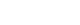a)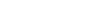b)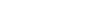c)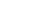d)Solution: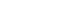=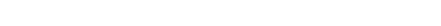Therefore the term independent of x is

==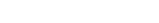=(c)

Our ISI CMI Entrance Program

Sequence Problem | ISI Entrance B.Math 2008 Obj 1 – Video

This is a problem from Test of Mathematics, TOMATO Problem 106. It is useful for ISI and CMI Entrance Exam. Try out the problem.

Problem: TOMATO Objective 106

The term that is independent of x in the expansion ofa)b)c)d)Solution:=Therefore the term independent of x is

===(c)

Our ISI CMI Entrance Program

Sequence Problem | ISI Entrance B.Math 2008 Obj 1 – Video

This site uses Akismet to reduce spam. Learn how your comment data is processed.

### Knowledge Partner